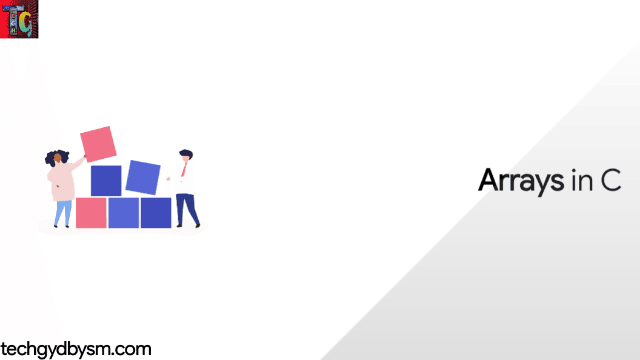# Arrays in C

Until now we've declared variables as individual quantity. But C offers us the facility of grouping data is similar types in a sequential format and store them in memory by an order. In this article we'll know about such data structures.Arrays in C

## Definition of array

The array is a data structure in C programming, which can store a collection of elements of same data type in a sequential pattern.Example of Array

For example, if you want to store ten numbers then instead of defining ten variables, it's easy to define an array of 10 lengths.

In C, an array can be One-Dimensional, Two-Dimensional and Multidimensional.

Any array of the syntax:

type arrayName[size];

falls under One-Dimensional Array. An array type is usually any valid C data type, but the array size must be an integer constant larger than zero.

## Declaration of array

We can declare an array by specifying either its type, size or by both.Array Declaration in C

### By specifying size:

``````// Array declaration by specifying size
int arr1;
int n=20;
int arr2[n]; /*this type of declaration is supported in latest c/cpp compilers*/``````

The first type of declaration instructs the compiler to allocate 5 memory units. The second type assigns an integer data type variable 'n' as 20. Then an array is declared that will call for 20 units in memory.

### By initialising elements:

``````int arr1[]={1,2,3,4};
int arr2={15,25,35,45};``````

The above array arr1 has 4 elements that are already declared alongside the data type of array. The array arr2 has different elements but also mentioned the number of elements in the [ ]. Both serve same purpose.

### By both specifying size and initialising elements:

Already mentioned in the above array arr2={15,25,35,45} where we have size specified and its elements initialised.

## Initialization of array:

In C, array can be initialised in either one by one or in a single statement as follows:

double sum={100.0,26.0,3.45,71.2,59.0};

The number of elements between braces { } cannot be greater than the number of elements that we have declared for the array between square brackets [ ].

But if the size of array is omitted, that array is big enough to hold the elements that are initialized. Therefore, if we write:

double sum[ ]={100.0,26.0,3.45,71.2,59.0};

We just created exactly the same array as we did in the previous example. In the following example, we will see how to assign a single element of an array:

sum=59.0;

The above statement assigns the 5th element in the array with a value of 59.0. 0 is the index of any array's first element and is also called the base index and the last index of any array is the total size of that array minus 1. In the following example we can see the array we have discussed above:Array Representation

## Accessing array elements:

Any element of a declared array can be accessed by indexing that array's name. For that task, we usually place the index position value in the [ ] which follows after the array's name. For example:

double salary=sum;

The above statement will fetch the 5th element from the array and assign the value to salary variable. The following example shows how to use all the above mentioned concepts viz. declaration, assignment, and accessing arrays −

``````#include<stdio.h>
int main()
{
int n; /*n is an array containing 5 integers*/
int i,j;
/*initialize elements of array n to 0*/
for(i=0;i<5;i++)
{
n[i]=i+100; /*set element at location i to i + 100*/
}
/*output each array element's value*/
for(j=0;j<5;j++)
{
printf("Element number [%d]=%d\n",j,n[j]);
}
return 0;
}``````

#### Output:Array Output in C

## Importance of array:

There is no issue in particular when we have lesser or countable number of data to input. But if we want to store large number of data, it is difficult to manage them by declaring normal variables. A very simple example of a small amount of data is "ages of family members". But if we are asked to find age of all members in a society, then the data size is very large. So many variables will only end up in confusion and delay in work. An array by label ageMembers and data type integer with array size 500 can simplify the task multiple folds.

All programs written in this post are compiled online.

### Books I Prefer:I hope this article was helpful to understand arrays in C! Comment below, if you've got any question. Head back soon for another interesting article on C Programming.